# Studying the effect of spin removal in the Ising model

0
553## Abstract

The Ising model is an exactly solvable statistical system at one or two dimensions that exhibits phase transitions and critical phenomena1. This paper explores the effect of the removal of lattice sites from the centre and the corners on the system being modelled. To simulate the Ising model, the Metropolis Algorithm is used. This paper will show that the number of sites and the location of the sites are important parameters, since sites at some locations have a higher interaction.

## Introduction

The Ising model is the simplest representation that can model the essential features of real systems, such as the critical point2.

The Ising model was proposed in the 1920s, and named after Ernst Ising. It is used to numerically solve the phase transitions that occur when changes in a certain parameter result in a large-scale change in the entire system being simulated.

The mathematical framework of the Ising model starts by creating a lattice that contains a set of equally spaced points. These points are referred to as lattice sites. Lattice sites share bonds, and if two sites are connected by a bond, they are called nearest neighbours. Ising models can be one-dimensional, two-dimensional, or three-dimensional.

Lattice sites are denoted by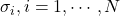; whereis the number of lattice sites in the system. Each sitetakes either of two values,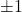, which represent two possible states of the site. The assignment of states (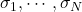) is referred to as a configuration of the system. Fig 1, 2, and 3 show lattices formed by their lattice sites in one-dimensional, two-dimensional, and three-dimensional Ising model respectively3.

Ernst Ising’s study focused on simulating ferromagnetism, as this paper will. In this system, at 2 or 3 dimensions, each lattice site represents an atom, which has a spin that can be either “up” or “down.” The net spin of the system determines its magnetic properties. The simulation used in this paper, however, focuses on the net spin itself and not the type of magnetic properties.

An important component of the Ising model is to find the total energy of a system, which is given by its Hamiltonian. For this, we assume that only two factors affect the total energy: (1) interactions of nearest-neighbour lattice sites and (2) interactions between an external field and the lattice sites4. The Hamiltonian is then given by

(1)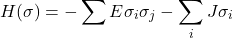where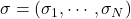. The first term is the summation over all nearest neighbours and E is a parameter corresponding to the energy associated with nearest-neighbour interactions. The second term is the summation over all lattice sites and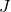is a parameter corresponding to external field interactions. The system prefers configurations with lower energy levels, and thus finding its energy is an essential component of simulating its equilibrium5.

Here,can be expressed as a product of two terms:

(2)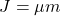Where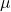is the atomic magnetic moment and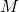is the strength of the external magnetic field. The Hamiltonian can then be expressed as

(3)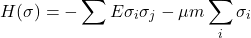The energy of the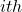atom,, can then be given by

(4)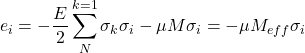Where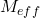is the effective magnetic field given by Drakos et al.6)

(5)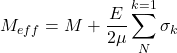In the model, the partition function, given by

(6)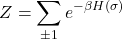where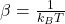;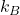is the Boltzmann’s constant and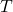is the temperature, and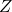is the summation over all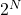possibleassignments to each lattice site, serves as a normalization constant for the probability of being in any given configurationover the Boltzmann distribution which is given by Kotze et al.7

(7)This probability also suggests that the probability of being in a state with a lower Hamiltonian (and thus energy) is greater than the probability of being in a state with a higher Hamiltonian, which means that the system prefers lower energy levels.

The Ising model is capable of showing the effect of magnitude of the parameters of the system: initial energy of the lattice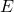, temperatureand thus also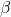, and the external field interactions as denoted by. As an example, it can show that average spin is close to 0 when temperature () is high and is closer to 1 when the temperature () is low. The Metropolis algorithm is covered in detail in the Results section.

This paper analyses the effect of the removal of lattice sites on the system, by observing the changes in the evolution of spin and energy, with parameters,andstaying constant.

## Results

It is difficult to manually mathematically solve the system since there arepossible configurations, in cases where no removal is used (and therefore there is no 0 state in any lattice site), whereis the number of lattice sites. For this reason, a simulation is used instead. The type of simulation chosen is Monte Carlo.

The Monte Carlo algorithm used is the Metropolis Algorithm, which is used to simulate a series of states of the system and reach an equilibrium. It starts with an initial lattice with a given size and configuration, along with its net spin and energy. The average spin is calculated by simply adding the spin of every individual lattice site and dividing it by the number of lattice sites. The net energy is calculated using the Hamiltonian. Then, it picks a random site in the lattice and proposes a flip in its spin (i.e., -1 to +1 or +1 to -1), by creating a temporary lattice with the spin reversed, and calculates the change in energy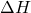that would be caused by the flip. If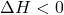, the spin flip is accepted, since the system prefers lower energy levels. If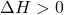, a random number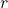is generated from the range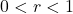. If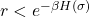, the spin flip is accepted. Else, it is rejected. If the proposed spin is accepted, the original lattice is overwritten by the temporary lattice, and the new net spin and energy of the lattice is stored. This process is repeated for a given number of Monte Carlo steps, until equilibrium is reached8. In this paper,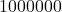Monte Carlo steps are used in our simulation on a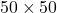lattice. In addition, the removal of lattice sites has been added to the metropolis algorithm before computing equilibrium. For this, given number of randomly chosen sites have their values set to 0. The code used for the simulation can be found on reference9.

First, the paper will analyze the effect of removing particles from the centre of the lattice on the evolution of average spin and net energy on a system with no external magnetic field. The size chosen is, and the centre was considered to be in the range of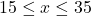and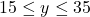. The values chosen for the parameters are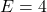and. No external magnetic field is considered. The parameter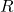represents the number of sites removed. The simulation was performed for a lattice initialized with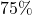of sites as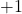(lattice p).

The results shown below are averages from 10 simulations.

The figures suggested that it might be possible to predict the average spin and net energy of the configuration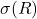, that is achieved at equilibrium whensites are removed, from their values at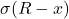where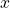is some constant. When removing sites from only the centre or outside the centre, a total of 400 sites were removed andwas chosen to be 10. When removing sites from the whole lattice, a total of 2000 sites were removed andwas chosen to be 50. A model was then trained on this data, and the accuracy with whichcould be predicted bywere then calculated, by using the R2 score of the model. The results are summarized in the table below:

The results suggest that the net energy ofcan be predicted by the net energy ofwith over 80\% accuracy when sites are removed from the whole lattice or the centre only, but with only 26\% accuracy when sites are removed from outside the centre only. In all cases, the average spin ofis not accurately predicted by the average spin of.

The percentage maximum variation of average spin and net energy when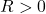,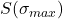andrespectively, from the average spin and net energy whenwas measured in all 3 cases.was not used for removal from the whole lattice as the larger values ofand the downward trend of net energy would skew the results. The results are shown below:

From these results we can observe that the location of the removed sites does have an impact, and sites seem to have different interactions. However,is not very different between except for when sites are removed from the whole lattice. This suggests that since sites in an area interact with each other, removing sites from a restricted area will have a larger impact than removing sites randomly.

## Discussion

While the Ising model undoubtedly has applications in theoretical and computational physics, its uses spread beyond that. D. Stauffer showed that the Ising model can compute socioeconomic systems, such as business confidence, racial segregation, and the replacement of the dominant language spoken in an area10). C. Daskalakis, N. Dikkala, G. Kamath also showed that the Ising model has applications in both synthetic and real-world social networks11). The results of this paper can perhaps be taken forward by applying removal of sites from such systems, representing exiting or temporary inactivity of agents in socioeconomics systems or social networks.

1. B. M. McCoy, J. Maillard, The importance of the Ising model. Progress of Theoretical Physics. 127, 791-817 (2012). []
2. S. G. Brush, History of the Lenz-Ising model. Reviews of Modern Physics. 39, 833 (1967). []
3. G. Ceder, A derivation of the Ising model for the computation of phase diagrams. Computational Materials Science. 1, 144-150 (1993). []
4. B. A. Cipra, An introduction to the Ising model. The American Mathematical Monthly. 94, 937-959 (1987). []
5. Y. Kozitsky, Order, Disorder and Criticality: Advanced Problems of Phase Transition Theory. 1-66 (2004). []
6. N. Drakos, R. Moore, The Ising model. https://farside.ph.utexas.edu/teaching/329/lectures/node110.html (2006 []
7. J. Kotze, Introduction to Monte Carlo methods for an Ising model of a ferromagnet. arXiv e-prints (2008). []
8. S. Jindal, Monte Carlo simulation of the Ising model. https://csc.ucdavis.edu/~chaos/courses/nlp/Projects2007/SaryuJindal/isingpaper.pdf (2007). []
9. P. Agrawal, Studying-the-effect-of-spin-removal-in-the-Ising-model. https://github.com/PraagyaAgrawal/Studying-the-effect-of-spin-removal-in-the-Ising-model (2023). []
10. D. Stauffer, Social applications of two-dimensional Ising models. American Journal of Physics. 76, 470-473 (2008 []
11. C. Daskalakis, N. Dikkala, G. Kamath, Concentration of multilinear functions of the Ising model with applications to network data. Advances in Neural Information Processing Systems. 30 (2017 []